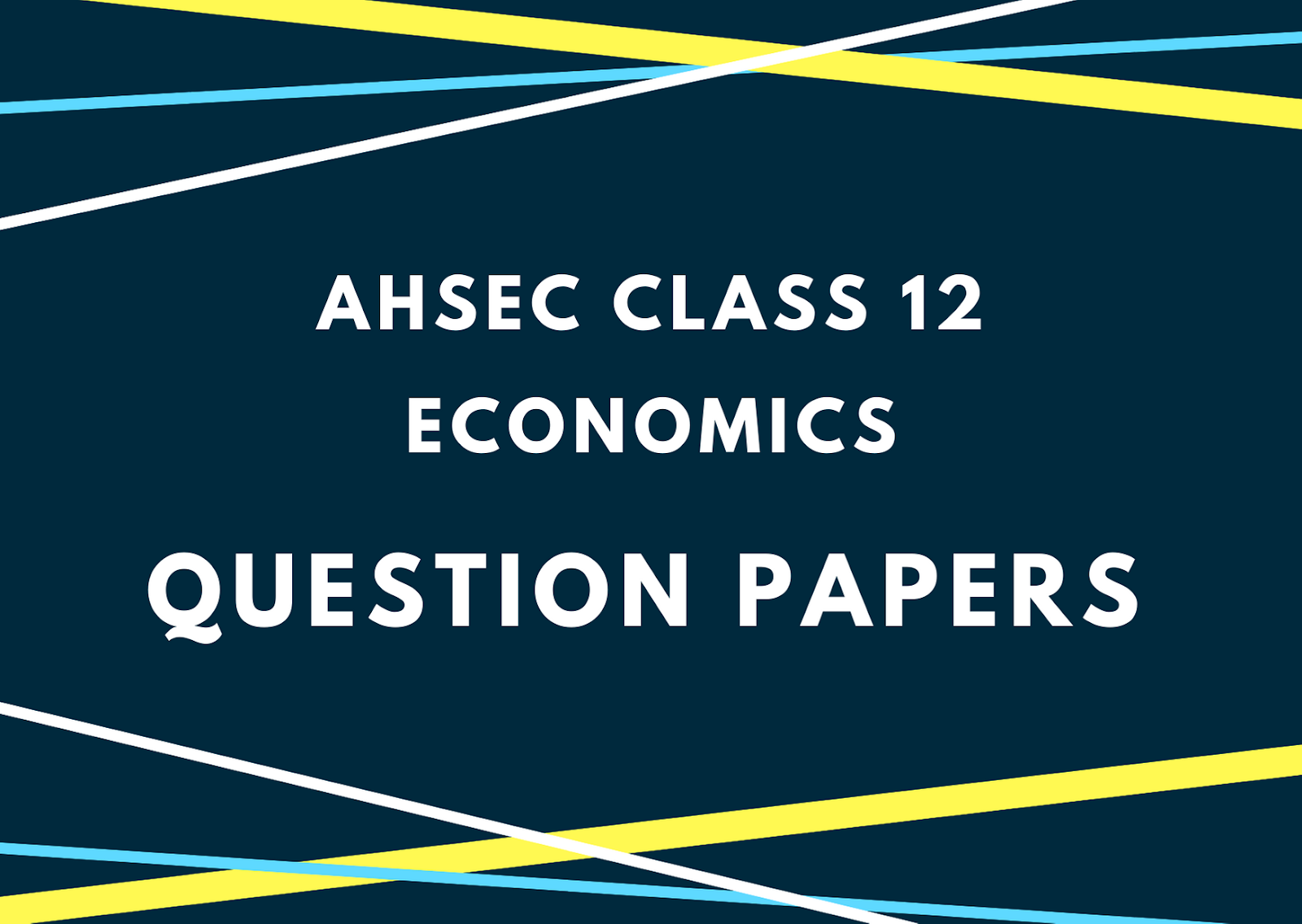## Sunday, December 09, 20182018
ECONOMICS
Full Marks: 100
Pass Marks: 30
Time: Three hours
The figures in the margin indicate full marks for the questions.
PART A

1. (a) Define market economy.                                  1
(b) What does a point below the production possibility frontier indicate?                          1
(c) What is meant by inferior goods?                   1
(d) What is an indifference curve?                       1
(e) If there is no change in quantity demanded despite a change in the price of a commodity, what will be the price elasticity (ep) of demand?                                            1
(f) What is a production function?                        1
2. Distinguish between positive economics and normative economics.                                   2
3. Mention any two important factors determining price elasticity of demand.                    2
4. Suppose, when the price of a good is Rs. 4, the quantity demanded is 25 units. As price increases to Rs. 5, the quantity demanded falls to 20 units. Calculate the price elasticity of demand.                    2
5. Can there be some fixed cost in the long run? Justify your answer.                                      1+1=2
Or
What is marginal product of an input?                                    2
6. Write down the concept of normal profit.                        2
7. What is monopolistic competition?                                     2
8. What does the Average Fixed Cost (AFC) curve look like? Why does it look so?              1+3=4
Or
What is meant by Returns to Scale? Give the meanings of various stages of Returns to Scale.   1+3=4
9. What is an isoquants? Why are the isoquants downward sloping?                                        2+2=4
10. What does a firm wish to achieve? What three conditions must hold for a profit maximising firm in the short-run? 1+3=4
Or
The production function of a firm is given as Q = L1/2K1/2. Calculate the level of output (Q) when it employs 25 units of labour (L) and 9 units of capital (K).                 4
11. Explain two characteristics of perfectly competitive market.                                       4
12. Explain the effects of a rightward shift of demand curve on equilibrium price and quantity when the number of firms in the market is fixed. Use suitable diagram for your answer.               4
13. What is demand curve? Show with the help of suitable diagrams how the market demand curve can be derived from individual demand curves.                                           1+5=6
Or
Explain consumer’s optimum with the help of indifference curve approach.                        6
14. The short run Total Cost (TC) schedule of a firm is given below. Calculate the TFC, TVC, AFC, AVC, AC and MC schedules of the firm.                                         6
 Q TC 0 1 2 3 4 5 10 30 45 55 70 90
Or
Give the meaning of Total Fixed Cost (TFC) and Total Variable Cost (TVC). Show with the help of a diagram that Total Cost (TC) is the sum of TFC and TVC.                                          2+4=6
PART B
15. (a) What is macroeconomics?                              1
(b) What is autonomous consumption expenditure?                 1
(c) Define excess demand in the context of income and employment determination.     1
(d) What are public goods?                                    1
(e) What is balanced budget?                               1
(f) Write down the meaning of flexible exchange rate.                             1
16. What is GDP Deflator?                            2
Or
Define budget deficit and trade deficit.                           2
17. What is Personal Disposable Income (PDI)?                  2
18. Distinguish between demand deposits and time deposits.                    2
19. What is the difference between Ex-ante investment and Ex-post investment?                 2
20. Mention any two items of non-plan expenditure of a government budget.                        2
21. What is Balance of Payment (BoP)? Mention two main accounts of BoP.                  1+1=2
22. Suppose the GDP at market price of a country in a particular year is Rs. 1100 crore. Net Factor Income from abroad is Rs. 100 crore. The value of Net Indirect Tax is Rs. 150 crore and National Income is Rs. 850 crore. Calculate the aggregate value of depreciation.                                4
23. Define Intermediate goods, Final goods, Consumption goods and Capital goods.                        4
24. Measure the level of ex-ante aggregate demand when autonomous investment and consumption expenditure Ȃ is Rs. 50 crore, MPS is 0.2 and level of income (Y) is Rs. 4,000 crore. State whether the economy is in equilibrium or not (cite reasons).                                                             3+1=4
Or
The autonomous consumption in an economy is Rs. 500 crore and total personal disposable income is Rs. 5,000 crore. If the marginal propensity to consume (mpc) is 0.8, find out the level of aggregate consumption.                          4
25. What is revenue deficit in a government budget? Explain its implications of revenue deficit.                                 1+3=4
26. Differentiate between devaluation and depreciation of currency.                                     4
27. Explain the circular flow of income in a simplified economy with two sectors – households and firms.                               6
Or
Write down some of the limitations of using GDP as an index of welfare of a country.        6
28. What are the main functions of money? How does money overcome the shortcomings of a barter system? 2+4=6
Or
Briefly explain any four functions of the RBI.                                                       6
***

#### 1 comment:

1.Thanks you so much sir

Kindly give your valuable feedback to improve this website.